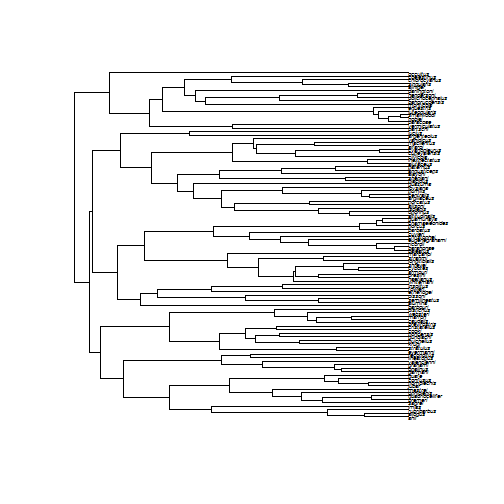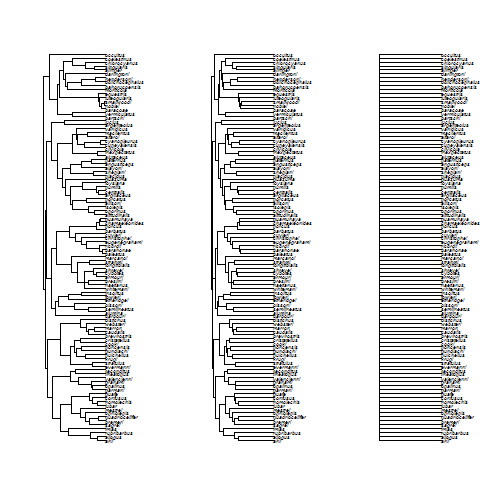## Exercise 5.1: Models of continuous character evolution

Let's fit some models of continuous character evolution. First, we will learn how to do some tests of “phylogenetic signal,” a very common test especially for ecological analyses. Then we will learn how to fit a series of evolutionary models for continuous characters.

``````library(geiger)
library(picante)
``````
``````## Warning: package 'picante' was built under R version 3.3.1
``````
``````## Loading required package: vegan
``````
``````## Warning: package 'vegan' was built under R version 3.3.1
``````
``````## Loading required package: permute
``````
``````## Warning: package 'permute' was built under R version 3.3.1
``````
``````## Loading required package: lattice
``````
``````## This is vegan 2.4-0
``````
``````## Loading required package: nlme
``````
``````library(phytools)
``````

We will use the following two data files of Anolis lizards:

If you need to, make sure these files are in your working directory and read them in.

``````anoleData <- read.csv("anolisDataAppended.csv", row.names = 1)
``````

If you have the data, then the following commands should work:

``````plot(anoleTree,cex=0.5)
````````````name.check(anoleTree, anoleData)
``````
``````##  "OK"
``````

Let's do the two main tests for phylogenetic signal using anole body size. The first test is Blomberg's K, which compares the variance of PICs to what we would espect under a Brownian motion model. K = 1 means that relatives resemble one another as much as we should expect under BM; K < 1 means that there is less “phylogenetic signal” than expected under BM, while K > 1 means that there is more. A significant p-value returned from phylosignal tells you that there is significant phylogenetic signal - that is, close relatives are more similar than random pairs of species.

``````anoleSize <- anoleData[, 1]
names(anoleSize) <- rownames(anoleData)
phylosignal(anoleSize, anoleTree)
``````
``````##          K PIC.variance.obs PIC.variance.rnd.mean PIC.variance.P
## 1 1.553678        0.1389386             0.7776082          0.001
##   PIC.variance.Z
## 1      -3.884389
``````
``````phylosig(anoleTree, anoleSize, method = "K", test = T)
``````
``````## \$K
##  1.553678
##
## \$P
##  0.001
``````

Another method for testing phylogenetic signal is Pagel's λ. λ is a tree transformation that stretches tip branches relative to internal branches, making the tree more and more like a complete polytomy. If our estimated λ = 0, then the traits are inferred to have no phylogenetic signal. λ = 1 corresponds to a Brownian motion model; 0 < λ < 1 is in between.

``````# First let's look at what lambda does
anoleTreeLambda0 <- rescale(anoleTree, model = "lambda", 0)
anoleTreeLambda5 <- rescale(anoleTree, model = "lambda", 0.5)

par(mfcol = c(1, 3))
plot(anoleTree)
plot(anoleTreeLambda5)
plot(anoleTreeLambda0)
````````````phylosig(anoleTree, anoleSize, method = "lambda", test = T)
``````
``````## \$lambda
##  1.016502
##
## \$logL
##  -3.810016
##
## \$logL0
##  -60.01946
##
## \$P
##  2.892589e-26
``````
``````lambdaModel <- fitContinuous(anoleTree, anoleSize, model = "lambda")
``````
``````## Warning in fitContinuous(anoleTree, anoleSize, model = "lambda"): Parameter estimates appear at bounds:
##  lambda
``````
``````brownianModel <- fitContinuous(anoleTree, anoleSize)
nosigModel <- fitContinuous(anoleTreeLambda0, anoleSize)

lambdaModel\$opt\$aicc
``````
``````##  15.65081
``````
``````brownianModel\$opt\$aicc
``````
``````##  13.52452
``````
``````nosigModel\$opt\$aicc
``````
``````##  124.1626
``````
``````# Conclusion: Brownian model is best, no signal model is terrible
``````

We can use `fitContinuous` to fit OU and EB models as well.

``````brownianModel <- fitContinuous(anoleTree, anoleSize)
OUModel <- fitContinuous(anoleTree, anoleSize, model = "OU")
EBModel <- fitContinuous(anoleTree, anoleSize, model = "EB")

# inspect results
brownianModel
``````
``````## GEIGER-fitted comparative model of continuous data
##  fitted 'BM' model parameters:
##  sigsq = 0.136160
##  z0 = 4.065918
##
##  model summary:
##  log-likelihood = -4.700404
##  AIC = 13.400807
##  AICc = 13.524519
##  free parameters = 2
##
## Convergence diagnostics:
##  optimization iterations = 100
##  failed iterations = 0
##  frequency of best fit = 1.00
##
##  object summary:
##  'lik' -- likelihood function
##  'bnd' -- bounds for likelihood search
##  'res' -- optimization iteration summary
##  'opt' -- maximum likelihood parameter estimates
``````
``````OUModel
``````
``````## GEIGER-fitted comparative model of continuous data
##  fitted 'OU' model parameters:
##  alpha = 0.000000
##  sigsq = 0.136160
##  z0 = 4.065918
##
##  model summary:
##  log-likelihood = -4.700404
##  AIC = 15.400807
##  AICc = 15.650807
##  free parameters = 3
##
## Convergence diagnostics:
##  optimization iterations = 100
##  failed iterations = 0
##  frequency of best fit = 0.75
##
##  object summary:
##  'lik' -- likelihood function
##  'bnd' -- bounds for likelihood search
##  'res' -- optimization iteration summary
##  'opt' -- maximum likelihood parameter estimates
``````
``````EBModel
``````
``````## GEIGER-fitted comparative model of continuous data
##  fitted 'EB' model parameters:
##  a = -0.736272
##  sigsq = 0.233528
##  z0 = 4.066519
##
##  model summary:
##  log-likelihood = -4.285970
##  AIC = 14.571939
##  AICc = 14.821939
##  free parameters = 3
##
## Convergence diagnostics:
##  optimization iterations = 100
##  failed iterations = 0
##  frequency of best fit = 0.42
##
##  object summary:
##  'lik' -- likelihood function
##  'bnd' -- bounds for likelihood search
##  'res' -- optimization iteration summary
##  'opt' -- maximum likelihood parameter estimates
``````
``````# calculate AIC weights
bmAICC <- brownianModel\$opt\$aicc
ouAICC <- OUModel\$opt\$aicc
ebAICC <- EBModel\$opt\$aicc

aicc <- c(bmAICC, ouAICC, ebAICC)
aiccD <- aicc - min(aicc)
aw <- exp(-0.5 * aiccD)
aiccW <- aw/sum(aw)
aiccW
``````
``````##  0.5353068 0.1848779 0.2798153
``````

It is important to realize that measurement error can bias your inferences with fitting these models towards OU. Fortunately, we can easily account for that in fitContinuous.

``````# We measured 20 anoles per species, and the standard deviation within each
# species was, on average, 0.05
seSize <- 0.05/sqrt(20)

# redo with measurement error
brownianModel <- fitContinuous(anoleTree, anoleSize, SE = seSize)
OUModel <- fitContinuous(anoleTree, anoleSize, model = "OU", SE = seSize)
EBModel <- fitContinuous(anoleTree, anoleSize, model = "EB", SE = seSize)

# calculate AIC weights
bmAICC <- brownianModel\$opt\$aicc
ouAICC <- OUModel\$opt\$aicc
ebAICC <- EBModel\$opt\$aicc

aicc <- c(bmAICC, ouAICC, ebAICC)
aiccD <- aicc - min(aicc)
aw <- exp(-0.5 * aiccD)
aiccW <- aw/sum(aw)
aiccW
``````
``````##  0.5345965 0.1846326 0.2807709
``````

### Challenge Problem 3: Fitting models of continuous character evolution

Estimate phylogenetic signal using Blomberg's K and Pagel's λ, the fit the BM, OU, and EB models to the data. The second column of the data matrix contains the standard errors of each species mean. Read the documentation files of `phylosig` and `fitContinuous` to figure out how that can be taken into account. Which model fits best?